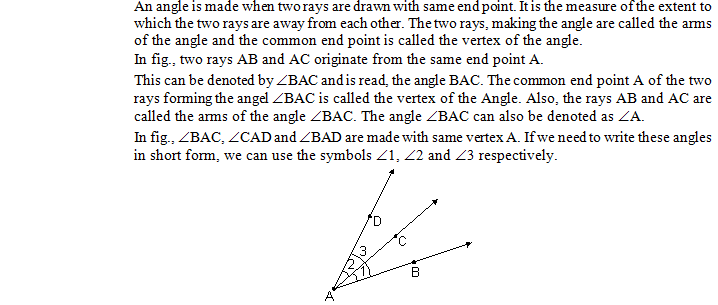# Angles and pairs of angles

## Lines and Angles of Class 7lines and angles, lines and angles class 9, class 7, angles, lines and angles class 7, lines and angles class 7 ncert, class,questions on lines and angles for class 7, lines, class 7 vii maths chapter 5 lines and angles, class 9 lines and angles shiksha house, lines and angles ncert, lines and angles questions, maths lines and angles, lines and angles solution, lines and angles worksheet